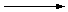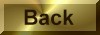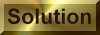Practice Problem 3

Use half-reactions to balance the equation for the reaction between sulfur dioxide and the dichromate ion in acidic solution.

Answer

Cr2O72-(aq) + 3 SO2(aq) + 2 H+(aq)2 Cr3+(aq) + 3 SO42-(aq) + H2O(l)